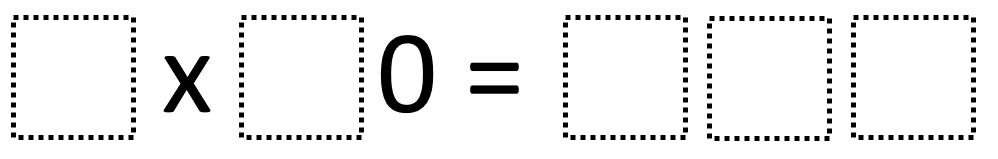# Multiplying Multiples Of Ten 1

Directions: Using the digits 0 to 9 at most one time each, place a digit in each box to make two different true number sentences: one with a product that’s less than 500 and one with a product that’s greater than 500. You may reuse all the digits each product.### Hint

How can you tell where the zero will go? How can your knowledge of multiplying one-digit numbers help you here?

There are many possible answers including 9 x 30 = 270 and 8 x 70 = 560

Source: Robert Kaplinsky

## Make The Time

Directions: Using the digits 0 to 9 at most one time each, place a digit …

1.9 x 80 = 720

2.7 x 50 = 350

3.2 x 50 = 100

4.one would be 6 x 80 = 480 and another could be 90 x 6 = 540

5.